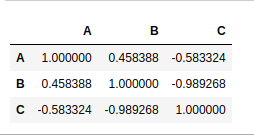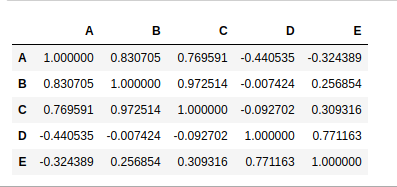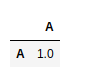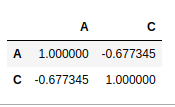# How to Create a Correlation Matrix using Pandas?

Correlation is a statistical technique that shows how two variables are related. Pandas dataframe.corr() method is used for creating the correlation matrix. It is used to find the pairwise correlation of all columns in the dataframe. Any na values are automatically excluded. For any non-numeric data type columns in the dataframe it is ignored.

To create correlation matrix using pandas, these steps should be taken:

1. Obtain the data.
2. Create the DataFrame using Pandas.
3. Create correaltion matrix using Pandas

Example 1:

 `# import pandas ` `import` `pandas as pd ` ` `  `# obtaining the data ` `data ``=` `{``'A'``: [``45``, ``37``, ``42``], ` `        ``'B'``: [``38``, ``31``, ``26``], ` `        ``'C'``: [``10``, ``15``, ``17``] ` `        ``} ` `# creation of DataFrame ` `df ``=` `pd.DataFrame(data) ` ` `  `# creation of correlation matrix ` `corrM ``=` `df.corr() ` ` `  `corrM `

Output:Values at the diagonal shows the correlation of a variable with itself, hence diagonal shows the correlation 1.

Example 2:

 `import` `pandas as pd ` ` `  `data ``=` `{``'A'``: [``45``, ``37``, ``42``, ``50``], ` `        ``'B'``: [``38``, ``31``, ``26``, ``90``], ` `        ``'C'``: [``10``, ``15``, ``17``, ``100``], ` `        ``'D'``: [``60``, ``99``, ``23``, ``56``], ` `        ``'E'``: [``76``, ``98``, ``78``, ``90``] ` `        ``} ` ` `  `df ``=` `pd.DataFrame(data) ` ` `  `corrM ``=` `df.corr() ` `corrM `

Output:Example 3:

 `import` `pandas as pd ` ` `  `# Integer and string values can  ` `# never be correlated. ` `data ``=` `{``'A'``: [``45``, ``37``, ``42``, ``50``], ` `        ``'B'``: [``'R'``, ``'O'``, ``'M'``, ``'Y'``], ` `        ``} ` ` `  `df ``=` `pd.DataFrame(data) ` ` `  `corrM ``=` `df.corr() ` `corrM `

Output:Example 4:

 `import` `pandas as pd ` ` `  `data ``=` `{``'A'``: [``45``, ``37``, ``42``, ``50``], ` `        ``'B'``: [``'R'``, ``'O'``, ``'M'``, ``'Y'``], ` `        ``'C'``: [``56``, ``67``, ``68``, ``60``], ` `               `  `        ``} ` ` `  `df ``=` `pd.DataFrame(data) ` ` `  `corrM ``=` `df.corr() ` `corrM `

Output:My Personal Notes arrow_drop_upCheck out this Author's contributed articles.

If you like GeeksforGeeks and would like to contribute, you can also write an article using contribute.geeksforgeeks.org or mail your article to contribute@geeksforgeeks.org. See your article appearing on the GeeksforGeeks main page and help other Geeks.

Please Improve this article if you find anything incorrect by clicking on the "Improve Article" button below.

Article Tags :

Be the First to upvote.

Please write to us at contribute@geeksforgeeks.org to report any issue with the above content.